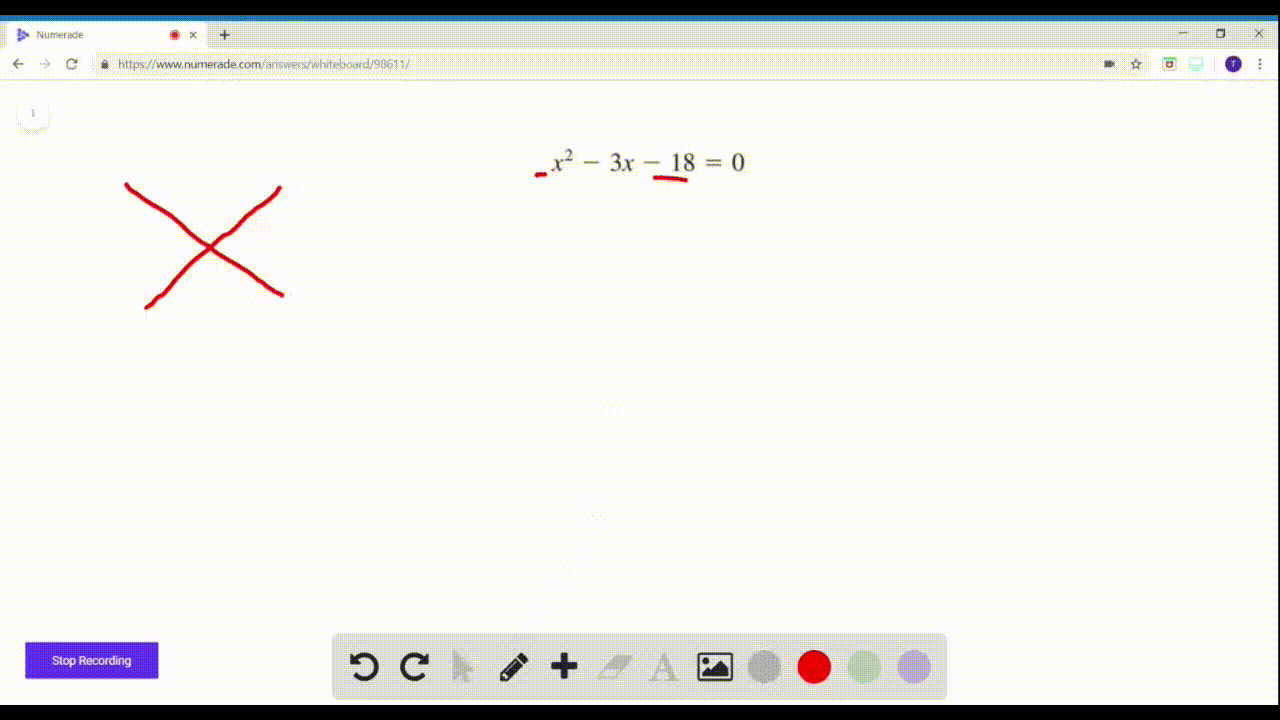Enroll in one of our FREE online STEM summer camps. Space is limited so join now!View Summer Courses### $29-46=$ Solving Quadratic Equations Find all rea…

02:02Central Michigan University

Need more help? Fill out this quick form to get professional live tutoring.

Get live tutoring
Problem 29

$29-46=$ Solving Quadratic Equations Find all real solutions of
the equation.
$$x^{2}-8 x+12=0$$

$x=\{2,6\}$

## Discussion

You must be signed in to discuss.

## Video Transcript

X squared minus eight X plus 12 equal zero we're gonna solve this equation is a quadratic equation and we're being told to just find all the solutions of the questions. So I'm left to assume we can use any method we want on the legal officials. One which makes me think wanted two things. The middle term is even too, which means completely square would be a good method. But maybe factoring are the factors of 12 that add up to negative eight and there are on negative, too, and negative. Six are factors of 12 that add up to negative eight so we can factor. This expression is X minus, two times X minus six and then we can use the zero product property to solve it. And we know that the answers are too, and six, because those are the values that would make these expressions equal to zero, making the whole entire expression zero. So using factoring on this one and zero product properly definitely the fastest way to go